# Graph Convolutional Networks (GCN) & Pooling

You know, who you choose to be around you, let’s you know who you are. — The Fast and the Furious: Tokyo Drift.

# What is the challenge?

In social networks, friend connections can be realized by a social graph.

In speech recognition, the phoneme Yᵢ and the acoustic model xᵢ form an HMM (a graph for speech recognition).

Even on CNN, an input image can be modeled as a graph. For example, the right diagram below is the graph for a 5 × 5 image. Each node represents a pixel and for the case of a 3 × 3 filter, every node is connected to its eight immediate neighbors.

Even though it is overkill to represent an image this way, a large percentage of machine learning (ML) problems will be much natural and effective to be modeled by a graph. In particular, when the relationships between neighboring nodes are irregular and high dimensional, we need to define them explicitly in order to solve them efficiently. In CNN, we work in a Euclidean space. How weights are associated with the input features (pixels) is well defined.

But this is not the case for a graph. For example, the graphs below are the same even though it looks different spatially.

In general, neural networks (NNs) takes an input x to predict z.

This leads us to the challenge of how a NN can process a graph directly.

In GCN (Graph Convolutional Network), the input to the NN will be a graph. Also, instead of inferring a single z, it infers the value zᵢ for each node i in the graph. And to make predictions for Zᵢ, GCN utilizes both Xᵢ and its neighboring nodes in the calculation.

Let’s detail it a little more.

# Graph Convolutional Networks (GCN)

The general idea of GCN is to apply convolution over a graph. Instead of having a 2-D array as input, GCN takes a graph as an input.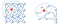Source

The first diagram (the first row) below is the NN as we know and the second diagram is the GCN with a graph containing four nodes as the input.

In the first NN, it contains multiple dense layers (fully connected layers). x is the input for the first layer and zᵢ is the output of layer i. For each layer, we multiple z (or x for the first layer) with the weight matrix W and pass the output to an activation function σ, say ReLU. GCN is very similar, but the input to σ is ÂHⁱWⁱ instead of Wᵢzᵢ. i.e. σ(Wᵢzᵢ) v.s. σ(ÂHⁱWⁱ) where zᵢ and Hⁱ are the output vectors from the last hidden layer for NN and GCN respectively. But please note that Wⁱ and Wᵢ are different and have different dimensions. And for the first layer in GCN, X contains an array of nodes instead of a single node x. X will be encoded as a matrix with each row contains the features of a node.

So what is Â? GCN introduces an adjacency matrix A. The element Aᵢⱼ in A equals 1 if node i and j are connected. Otherwise, it will be zero. So Â indicates the neighbors of a node. But we will make one more adjustment to indicate all nodes are self-connected. This indicates the output of a node in a hidden layer depends on itself and its neighbors. So, we convert all diagonal elements of A to 1 to form Â. Mathematically, Â equals A + I.

That comes to the output of the hidden layer to be σ(ÂHⁱWⁱ). If we ignore W for a second, for each node in a hidden layer, ÂHⁱ sums up features on each node with its neighbors.

However, we may face the diminishing or exploding problem in a NN if we don’t have certain control over the range of the hidden layer output. In specific, GCN wants Â to be normalized to maintain the scale of the output feature vectors. One possibility is to multiple Â with D̂⁻¹ where D̂ is the diagonal node degree matrix of Â in measuring the degree of each node. At a high level, instead of summing up itself with its neighbor, multiplying the sum with the inverse D̂⁻¹ sort of averages them. Specifically, D̂ is a diagonal matrix with each diagonal element D̂ᵢᵢ counts the number of edges for the corresponding node i. And the output for each hidden layer becomes σ(D̂⁻¹ÂHⁱWⁱ), instead of σ(ÂHⁱWⁱ).

Let’s calculate D̂ in our example. For an undirected graph, the degree of a node is counted as the number of times an edge terminates at that node. So a self-loop will count twice. In our example, node 0 has 2 edges connecting to its neighbors plus a self-loop. Its degree equals 4 (i.e. 2 + 2). For node 3, its degree equals 5 (3 + 2).

And D̂⁻¹ equals

The diagram below summarizes the model discussed so far. In this example, it has 3 hidden layers and for each hidden layer, it computes its output as σ(D̂⁻¹ÂHⁱWⁱ). The equation used to compute a hidden layer output from the last layer output is called the propagation rule.

Besides using σ(D̂⁻¹ÂHⁱWⁱ), there are other choices. Indeed, the propagation rule can be generalized as:

Different choices of f result in different variants of the models. As a preview, the GCN paper applies the propagation rule below

Â and D̂⁻¹ are calculated in the same way as before. But let’s defer the discussion later and work on an example instead. Let’s demonstrate it with Zachary’s karate club network problem.

Zachary’s karate club

There is a karate club that has two major stakeholders: the instructor (Mr. Hi) and the administrator. Unfortunately, the dispute between them causes it to split into 2 clubs. The original members will need to choose a side and pick which one to join. Their decisions will be based on how well they are connected with Mr. Hi or the administrator. This also includes how well they are connected to members that are associated with them. The diagram below is the social graph in representation connections between members.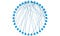Source: Wikipedia (The member # start from 1)

Let’s organize the graph a little bit more with blue and red being the ground truth of which club a member will join (Mr. Hi’s club is marked with red color below).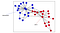The member # start from 0

To find the club membership, we apply two hidden layers below with Hⁱ⁺¹ = σ(D̂⁻¹ÂHⁱWⁱ). The input will be the social graph above (without the club labels) and the last hidden layer will be the output.

The last hidden layer outputs 2 scalar latent features per node and we use these values to represent a node. The nice part is, in this example, we can compute the latent representation based on the social graph alone even without training. We run the model for one iteration with W⁰ and initialized randomly with a normal distribution. But we are not going to perform gradient descent to update W. For X, we simply use an identity matrix as there is no node feature (as we have no prior knowledge of these features).

In the end, we plot out each node using the output latent features as the x and y coordinates.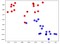This model is using σ(D̂⁻¹ÂHⁱWⁱ) as the propagation rule

As shown, when we color each node with its ground truth (blue for Mr. Hi club and red for the administrator), the latent features generated are highly correlated with which club a person belongs to. In short, we just apply clustering on the input social graph. Intuitively, our example produces latent features similar to their neighbors as the hidden layer is averaging the latent features with its neighbors. The algorithm manages to cluster neighbors together by generating latent factors related to their neighbors.

Let’s consider a semi-supervised problem for classification or clustering in which only one data point is labeled for each class or cluster. Once the latent features for each node are calculated, we can compute the distance between non-labeled data and the labeled data. Then, we find the nearest neighbor to a known labeled data to classify or cluster the unlabeled data.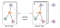Source

All propagation rules discussed so far are differentiable. For semi-supervised or unsupervised problems, we can use backpropagation to train the weights using the labeled data if needed. (For our last example, even without the extra training, the result is good.) As a demonstration, the GCN model below is trained for 300 iterations. In this model, only a single label per class is provided. As the training progress, we see how nodes belonging to the same ground truth classes start clustering together.

# Spectral Graph Convolution

Engineering problems can be solved in the spatial domain or the spectral domain (a.k.a. frequency domain). For example, in signal processing, we apply a Fourier transform to convert an audio input from the temporal domain to the frequency domain and apply a low-pass filter to remove the high-frequency noise. In convolution, these filters can be defined with a spatial or a spectral approach. Which domain to use for the specific step depends on how easy to design the filter and how easy to perform the operation.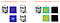Source

In deep learning, researchers also approach the convolution from either the spatial or spectral-domain. For example, in the previous section, we apply a spatial graph convolution in the form of σ(D̂⁻¹ÂHⁱWⁱ).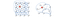Source

But GCN is actually a spectral graph convolution. It is a localized first-order approximation of spectral graph convolutions with the propagation rule below.

The journey leading to this new propagation rule can be very long. But it is not very important to understand it thoroughly to use it. In particular, the new rule is quite similar to the one before. We just add more mathematical justification. Feel free to browse through the next two sections quickly.

# Spectral Graph Convolution

However, it will need some mathematical concepts, equations, and a few research papers to explain a spectral graph convolution. And because of its complexity, we will show the high-level steps only.

Convolutional filters are translation-invariant to allow weight sharing (the same filter slides over an input). When working in the spatial domain, it recognizes identical features independently of their spatial locations. A graph does not have a clear spatial concept or a mathematical definition of spatial translation. This leads to questions on what is the mathematical foundations that spatial graph filters are based on.

On the other hand, the spectral graph convolution is based on spectral graph theory. It provides a mathematical framework to design operators (filters) with the translation-invariant property. This does not imply the spatial graph filters are non-scientific. But sometimes, they may fall back to empirical results for justifications.

At a high level, a spectral graph convolution is defined in the Fourier domain by applying the filter gθ on the input signal x.

Previously, we model a graph with the adjacency matrix A and normalize it with the diagonal node degree matrix D. Under the spectral graph theory, an undirected graph is represented by the normalized graph Laplacian matrix L which is defined as

L is a real symmetrical positive semidefinite matrix. But for an N×N matrix, if it is positive semidefinite, it has N orthogonal eigenvectors. (We will skip the proof here.) In short, these N independent vectors form a basis that can diagonalize a matrix, i.e., L can be factored as L = UΛUᵀ like the one below where U contains the eigenvectors of L and Λ is a diagonal matrix containing the corresponding eigenvalues. These eigenvectors are also known as the graph Fourier modes, and the corresponded eigenvalues are the frequencies of the graph.

The Laplacian is diagonalized by the Fourier basis U and the spectral convolution will be defined as a linear operator with U. In specific, the graph Fourier transform on x is defined as

Here, the graph convolution operator is defined in the Fourier domain. It is the multiplication of the graph x with a filter in the Fourier space. i.e.

x *G g means performing spectral convolution on x with filter g.

If we denote a filter as

Then the graph convolution can be simplified as:

Spectral Convolutional Neural Network assumes the filter gθ is a diagonal matrix filled with learnable parameters Θᵏᵢⱼ. Θᵏᵢⱼ contains parameters for layer k that maps the ith input feature to the jth output feature. For layer k, the output of a hidden layer output is

This is the mathematical framework for a spectral graph convolution.

The shortfall of Spectral Graph Convolution

While the spectral graph theory has operators with well-defined translation properties, computing the eigenvectors of L is computation expensive. We want to avoid that. Another shortfall is that these filters are not localized in general. We may not visit the kth closest neighbors for each node only. Its complexity grows with the size of the graph and not scalable. Indeed, to compute the output of a node efficiently, we should just hop over a limited number of neighbors, say at most K hops from the central node. This leads to researches of applying approximation to the spectral graph convolution, like those in ChebNet and GCN, such that filters are localized. And because of this issue, spatial graph convolution also receives more attention in recent years.

ChebNet

So how can we find a localized filter in the spectral graph convolution? Chebyshev Spectral CNN (ChebNet) approximates the filter gθ using a truncated expansion in terms of Chebyshev polynomials Tk(x) up to Kth order.

The convolution of a graph x with the defined filter gθ becomes:

This is K-localized as it is a Kth-order polynomial in the Laplacian. It depends only on nodes that are at maximum K steps away from the central node (details). It means it is localized in space and scales well regardless of the graph size. Even ChebNet and GCN start as a Spectral Graph Convolution, they apply approximation such that their filters are localized.

As Tᵢ(L̂) can be written as:

The convolution becomes

Hence, we don’t need to calculate the eigenvectors and it is localized.

# GCN

GCN introduces a first-order approximation of ChebNet by assuming K = 1 and λmax = 2. The equation becomes

To reduce the number of free parameters and to avoid over-fitting, GCN assumes θ = θ₀= −θ₁, and the equation becomes

But the L.H.S. term below has eigenvalues in the range [0, 2], to avoid exploding/vanishing problems, a renormalization trick is applied that results in the R.H.S. term below.

Finally, the propagation rule for GCN is

(Equation credits in this section: Source 1 and 2.)

Next, we will move onto Spatial Graph convolution.

# Spatial Graph Convolution

Different spatial graph convolutions depend on different aggregators to gather information from each node’s neighbors. Conceptually, we can also view it as message passing.

Without the approximations in Spectral Graph Convolutions, Spatial Graph Convolutions are usually more scalable since their filters are localized. The major challenge is defining a local invariance of CNNs that work with central nodes that have different numbers of neighbors. In the next few sections, we will overview different Spatial Graph convolution methods quickly. We may present equations that it may take sometimes for you to connect the dots. But not to lengthen the article further, please refer to individual papers for details.

Message Passing Neural Network (MPNN)

MPNN outlines a general message passing framework for Spatial Graph Convolution. It passes information (message) from one node to another along edges and repeats it K-step to let information propagate through the graph. The equation below is the hidden feature representation for the kth layer for node v. It depends on the hidden feature of v and its neighbors in the previous layer as well as the edge features with its neighbors. Different choices of U and M functions will lead to different variants of the model.

The latent representation of a node in the last hidden layer can be passed to an output layer to perform node-level predictions. Or it can pass to a readout function R with learnable parameters to perform graph-level predictions.

For example, in drug discovery, a graph represents a chemical compound with atoms as nodes and chemical bonds as edges. We may want to classify whether this compound can hinder the growth of cancer cells or it is carcinogenic. Therefore, we can perform a readout with the equation above in making a graph-level prediction.

Diffusion Convolutional Neural Network (DCNN)

DCNN treats graph convolutions as a diffusion process. It transfers information from one node to one of its neighbors with a probability transition matrix P that equals D⁻¹A. This process will repeat K times so that information distribution will reach an equilibrium. And the hidden representation Hat the kth step will be calculated from Pᵏ, but not on the last hidden representation.

DCNN concatenates H⁽¹⁾, H⁽²⁾, · · ·, H⁽ᴷ⁾ together to form the final model outputs.

Graph Isomorphism Network (GIN)

However, GIN claims that the graph embeddings in MPNN methods fail to distinguish different graph structures. A good scheme should distinguish different graph structures and map them to different latent features in the embedding space. To fix that, GIN introduces a learnable parameter εᵏ to adjust the weight of the central node.

GraphSage

Social influencers have many connections. For a graph with nodes that have many edges, convolutions may not scale well. GraphSage uses sampling to obtain a fixed number of neighbors for each node for message passing instead of all neighboring nodes.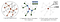Source

Here is the formularization:

Alternatively, we can compute an aggregated value for the neighbors first. For example, we can use an LSTM aggregator to compute a representation of the neighbors. Then we concatenate the values with the central node and apply a dense layer. Otherwise, we can apply W to all neighbors followed by an activation function and a max pool.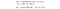Source

Sampling

FastGCN refines the sampling algorithm further. Instead of sampling neighbors for each node, FastGCN uses importance sampling to reduce variance. It samples nodes (u) from a distribution q that reflects how well it is connected to other nodes. Then, it applies importance sampling to estimate the loss gradient of node v from u.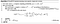Source

Graph Attention Network (GAT)

GAT uses attention to learn the relative weights between two connected nodes in message passing. First, GAT calculates the attention for the node pair i and j. The resulting vectors Whᵢ (hᵢ is the hidden representation of node i) and Whⱼ are concatenated (|| operator) and multiply with learnable vector a. Their attention, measuring the connective strength between them, is then computed with a softmax function.

The hidden representation for node i is then weighted by the computed attention α with the final result as

Mixture Model Network (MoNet)

MoNet uses node pseudo-coordinates to determine the relative position between a node and its neighbor. Consider node y is a neighbor of node x. First, a d-dimensional vector of pseudo-coordinates u(x, y) is calculated as below. The MoNet paper uses the degrees of the nodes (number of connection) as the pseudo-coordinates and then transforms them further with a fully-connected network.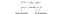Modified from source

Once the relative position between two nodes is calculated, a weight function maps the relative position to the relative weight between these two nodes. This weighting function w is parametrized by learnable parameters Θ and composed of J components:

In MoNet, the weight function is defined as:

where μⱼ and Σ are trainable parameters for a Gaussian.

Let’s summarize it. The coordinate system (x, y) for an image is defined in Euclidean space with the output pixel value being f(x, y). With MoNet, the weight function above allows us to expand the concept to non-Euclidean space and apply a shared filter that works across different locations. Below is the detailed equation for applying the convolution filter g on f.

MoNet can be generalized with different choices of u and weight functions. The diagram below shows how different GNN can be viewed as MoNet using the specific u and w.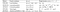Source

Large-Scale Learnable Graph Convolutional Networks (LGCN)

In each LGCL layer, it selects and sorts the largest k values for each input feature channel for the neighbors of the central node. With the features of the central node, it forms a (k+1) × 3 matrix. Then a CNN filter is applied. In the diagram below, two filters are applied to create a new node representation with 5 output channels.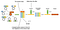Modified from source

LGCN applies multiple layers of LGCL as in the diagram below.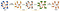Source

The input nodes are transformed into low-dimensional representations using a graph embedding layer. In some cases, it can simply use a linear transformation like the one below.

Then it follows with two LGCL layers with skip concatenation connections to produce the output, i.e. the inputs and outputs of LGCL are concatenated together. Finally, a fully-connected layer is used to classify each node.

# Pooling

In the diagram below, we apply layers of convolutions and ReLU to generate the latent representation for each node.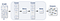Source

But in other applications, we are interested in generating a representation of the graph. For example, consider a sample (G, y) where G is a graph, and y is its class. As a classification problem, we read G and predict y — the representation of the graph. In this context, pooling can be applied to generate a coarser graph which can be further reduced with a readout operation. This is similar to the conventional CNN where we apply max pool to reduce the spatial resolution.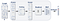Source

In general, the pooling and the readout are similar and mathematically, can be generalized as:Source

DGCNN

If there is a consistent way to map nodes in a graph to a Euclidean space, there will be no ambiguity on which weight should be associated with a specific node when a filter is applied. So for a spatial graph convolution, the challenge is how to assign weights to nodes consistently.

The two graphs below are similar in topology with the exception of the two red edges below. We color nodes in both graphs with similar colors if they have similar network topology. For example, both nodes D and 2 are colored as orange.

And when we apply a convolution filter, both graphs should generate similar latent features for the corresponding nodes. One way to do it is to sort nodes by the assigned color (say, by RGB values) and assign the corresponding weights accordingly.

DGCNN addresses the same problem on how to sequentially read a graph and associate weights in a consistent order. So it introduces the SortPooling layer which sorts graph nodes by color so that neural networks can be trained on these ordered nodes.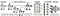Source

By presenting graphs in a consistent way according to their topology, the 1-D convolution and dense layers will be much easier to train. For example, the graphs below are isomorphic and will be presented in the same way to the NN after the SortPooling layer.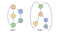Source

So how can we color nodes in DGCNN? It uses the Weisfeiler-Lehman algorithm.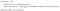Source

The concept is very similar to our first clustering example for Zachary’s karate club. But we will not get into the details here. Please refer to the DGCNN paper. Basically, it learns the network embedding (signature) of the nodes based on the network topology. It concatenates a node’s color with its immediate neighbors and then sorts it lexicographically to assign new colors. Nodes with the same signature will be assigned with the same color. The process is repeated until the colors converge or after h iterations. In the end, nodes with the same color share the same structural role within the graph. Then the colors in the last convolution layer will be used to sort the nodes. Therefore, a consistent ordering is imposed for graph nodes. After sorting, DGCNN truncates or extends the array of nodes. This supports graphs with different numbers of nodes.

DiffPool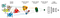Source

DiffPool is based on a hierarchical concept in generating a coarser graph. It learns a soft cluster assignment matrix Sˡ with element i, j contains the probability for the node i in layer l to be clustered and assigned to node j in the next hierarchical layer in a coarser graph.

Then, this soft assignment can be used to calculate the new node embedding and adjacency matrix for the next hierarchical layer.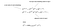Source for the equations

With Sˡ generated by

This model is differentiable and the objective is to learn both GNN models that generate Z and S.

# Credits and References

Graph Convolutional Networks

Semi-Supervised Classification with Graph Convolutional Networks

Convolutional Neural Networks on Graphs with Fast Localized Spectral Filtering

Math Behind GCN

Graph Convolutional Networks on GitHub

A Comprehensive Survey on Graph Neural Networks

Graph Neural Networks: A Review of Methods and Applications

An End-to-End Deep Learning Architecture for Graph Classification

Machine Learning with Graphs

Benchmarking Graph Neural Networks

Convolutional Neural Networks on Graphs with Fast Localized Spectral Filtering

Spectral Networks and Deep Locally Connected Networks on Graphs

Deep Learning